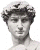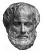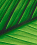xTrigonometry
Where does trigonometry usually apply?
Posts  1 - 3  of  3tbone
Where does trigonometry usually apply?
replied to:  tboneDamian
Replied to:  Where does trigonometry usually apply?
Trigonometry usually applies to pure mathematics and applied mathematics
replied to:  Damiangalaxaura
Replied to:  Trigonometry usually applies to pure mathematics and applied mathematics
There is also spherical trigonometry in which triangles inside squares is studied